##### Question

In: Statistics and Probability

# Let x be a random variable that represents the level of glucose in the blood (milligrams...

## Solutions

##### Expert Solution

Solution :

Given that ,

mean =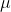= 51

standard deviation =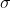= 47

a) P(x < 40) = P[(x -) /< (40 - 51) / 47]

= P(z < -0.23)

Using z table,

= 0.4090

b) n = 2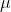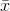== 51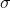=/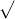n = 47/2 = 33.23

The probability distribution of x is approximately normal with μx = 51 and σx = 33.23

P(< 40) = P((-) /< (40 - 51) / 33.23)

= P(z < -0.33)

Using z table

= 0.3707

c) n = 3== 51=/n = 47/3 = 27.14

The probability distribution of x is approximately normal with μx = 51 and σx = 27.14

P(< 40) = P((-) /< (40 - 51) / 27.14)

= P(z < -0.41)

Using z table

= 0.3409

d) n = 5== 51=/n = 47/5 = 21.02

The probability distribution of x is approximately normal with μx = 51 and σx = 21.02

P(< 40) = P((-) /< (40 - 51) / 21.02)

= P(z < -0.52)

Using z table

= 0.3015

e) yes,

The more tests a patient completes, the weaker is the evidence for excess insulin

## Related Solutions

##### Let x be a random variable that represents the level of glucose in the blood (milligrams...
Let x be a random variable that represents the level of glucose in the blood (milligrams per deciliter of blood) after a 12-hour fast. Assume that for people under 50 years old, x has a distribution that is approximately normal, with mean μ = 88 and estimated standard deviation σ = 28. A test result x < 40 is an indication of severe excess insulin, and medication is usually prescribed. (a) What is the probability that, on a single test,...
##### Let x be a random variable that represents the level of glucose in the blood (milligrams...
Let x be a random variable that represents the level of glucose in the blood (milligrams per deciliter of blood) after a 12 hour fast. Assume that for people under 50 years old, x has a distribution that is approximately normal, with mean μ = 96 and estimated standard deviation σ = 47. A test result x < 40 is an indication of severe excess insulin, and medication is usually prescribed. (a) What is the probability that, on a single...
##### Let x be a random variable that represents the level of glucose in the blood (milligrams...
Let x be a random variable that represents the level of glucose in the blood (milligrams per deciliter of blood) after a 12 hour fast. Assume that for people under 50 years old, x has a distribution that is approximately normal, with mean μ = 84 and estimated standard deviation σ = 29. A test result x < 40 is an indication of severe excess insulin, and medication is usually prescribed. (a) What is the probability that, on a single...
##### Let x be a random variable that represents the level of glucose in the blood (milligrams...
Let x be a random variable that represents the level of glucose in the blood (milligrams per deciliter of blood) after a 12 hour fast. Assume that for people under 50 years old, x has a distribution that is approximately normal, with mean μ = 60and estimated standard deviation σ = 32. A test result x < 40 is an indication of severe excess insulin, and medication is usually prescribed. (a) What is the probability that, on a single test,...
##### Let x be a random variable that represents the level of glucose in the blood (milligrams...
Let x be a random variable that represents the level of glucose in the blood (milligrams per deciliter of blood) after a 12 hour fast. Assume that for people under 50 years old, x has a distribution that is approximately normal, with mean μ = 86 and estimated standard deviation σ = 35. A test result x < 40 is an indication of severe excess insulin, and medication is usually prescribed. (d) Repeat part (b) for n = 5 tests...
##### Let x be a random variable that represents the level of glucose in the blood (milligrams...
Let x be a random variable that represents the level of glucose in the blood (milligrams per deciliter of blood) after a 12-hour fast. Assume that for people under 50 years old, x has a distribution that is approximately normal, with mean μ = 71 and estimated standard deviation σ = 30. A test result x < 40 is an indication of severe excess insulin, and medication is usually prescribed. (a) What is the probability that, on a single test,...
##### Let x be a random variable that represents the level of glucose in the blood (milligrams...
Let x be a random variable that represents the level of glucose in the blood (milligrams per deciliter of blood) after a 12 hour fast. Assume that for people under 50 years old, x has a distribution that is approximately normal, with mean μ = 56 and estimated standard deviation σ = 42. A test result x < 40 is an indication of severe excess insulin, and medication is usually prescribed. A.) What is the probability that, on a single...
##### Let x be a random variable that represents the level of glucose in the blood (milligrams...
Let x be a random variable that represents the level of glucose in the blood (milligrams per deciliter of blood) after a 12 hour fast. Assume that for people under 50 years old, x has a distribution that is approximately normal, with mean μ = 56 and estimated standard deviation σ = 24. A test result x < 40 is an indication of severe excess insulin, and medication is usually prescribed. (a) What is the probability that, on a single...
##### Let x be a random variable that represents the level of glucose in the blood (milligrams...
Let x be a random variable that represents the level of glucose in the blood (milligrams per deciliter of blood) after a 12 hour fast. Assume that for people under 50 years old, x has a distribution that is approximately normal, with mean μ = 51 and estimated standard deviation σ = 47. A test result x < 40 is an indication of severe excess insulin, and medication is usually prescribed. (a) What is the probability that, on a single...
##### Let x be a random variable that represents the level of glucose in the blood (milligrams...
Let x be a random variable that represents the level of glucose in the blood (milligrams per deciliter of blood) after a 12 hour fast. Assume that for people under 50 years old, x has a distribution that is approximately normal, with mean μ = 52 and estimated standard deviation σ = 10. A test result x < 40 is an indication of severe excess insulin, and medication is usually prescribed. (a) What is the probability that, on a single...